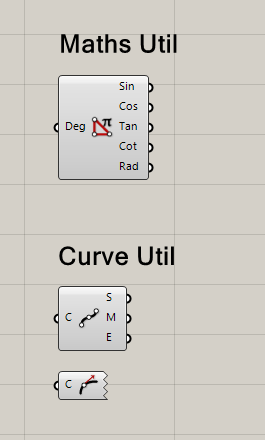# Code to create an arrow preview in GhPython

There is another problem in the last script ; the arrows appear but the curve are not appear in grasshopper
emm thats wird ; it work in a new document

I haven’t tested the script, but it also needs to compute the bounding box of all the geometry that it wants to preview. Then, the bounding box must be returned in the `get_ClippingBox` method. As it is shown here:

I moved this sub-topic to a new topic.

1 Like

Many thanks Giulio, i appreciate your help
i study now when many curves selected

1 Like

My Brain stopped herefrom ghpythonlib.componentbase import executingcomponent as component
import System
import rhinoscriptsyntax as rs
import Rhino.Geometry as rg

class PreviewEx(component):

``````def RunScript(self,C):
self.crv = C
if not self.crv: return
self.points = []
self.tgnt = []
for i in range(len(C)):
pts = rs.DivideCurve(self.crv[i],2,True)
for j in range(3):
pt = rs.CurveClosestPoint(self.crv[i],pts[j])
tg = rs.CurveTangent(self.crv[i],pt)
self.tgnt.append(tg)
self.points.append(pts[j])
#print self.points
#print self.tgnt

def DrawViewportWires(self,arg):

if not  self.crv: return
for i in range(len(self.crv)):
for i in range(3):
arg.Display.DrawDirectionArrow(self.points[i], self.tgnt[i], System.Drawing.Color.White)
``````

the input have two curves (or more )
in def DrawViewportWires(self,arg): the length of curves list have no effect
maybe because i append all points and tangents

I compile the script and it work fine with single curve without bounding box

One day it might not work fine. This is a requirement that does not show sometimes. But then when it shows, it is really bad. I also don’t see the point of compiling if the code is already online…
My 2 cents.

1 Like

I compile it to try if it work because sometimes the scripts it work fine but after compiling nope.
and of course i need the best solution that why i asked and i don’t really understand the idea of the bounding box, i will study it more.
I use always examples from help but some of them don’t work or need more lines to work

The bounding box computation is because of this: Rhino tries to optimize preview by using bounding boxes. If the thing that you want to draw is outside the “view frustum”, then its drawing function will not be called. This operation is called “view frustum culling”.

1 Like

Thank you ,I tried it but bounding box union don’t accept the points

Update:

i use this ; i don’t know if this work or not

i hope this ok, thanks for help Giulio

``````from ghpythonlib.componentbase import executingcomponent as component
import Grasshopper, GhPython
import System
import Rhino
import rhinoscriptsyntax as rs
import Rhino.Geometry as rg

class DisplayDirection(component):

def __init__(self):
self.pts = []
self.bb = Rhino.Geometry.BoundingBox()

def RunScript(self,C):
self.c = C
if not self.c: return
self.bb = Rhino.Geometry.BoundingBox()
self.tps = []
self.tgnt = []
self.pts = rs.DivideCurve(self.c,2,True)
for i in range(len(self.pts)):
self.bb.Union(self.pts[i])
self.pts.insert(2, rs.CurveEndPoint(self.c))

for i in range(3):
pt = rs.CurveClosestPoint(self.c,self.pts[i])
self.tps.append(pt)
tg = rs.CurveTangent(self.c,self.tps[i])
self.tgnt.append(tg)

def DrawViewportWires(self,arg):

if not  self.c: return
for i in range(3):
arg.Display.DrawDirectionArrow(self.pts[i], self.tgnt[i], System.Drawing.Color.White)

def get_ClippingBox(self):
return self.bb``````

Update: this version work with multi curves, i also add the idea of bounding box
i know there is always more simple method but that what i get

``````from ghpythonlib.componentbase import executingcomponent as component
import Grasshopper, GhPython
import System
import Rhino
import rhinoscriptsyntax as rs

class CurvesDirection(component):
def __init__(self):
self.pts = []
self.bb = Rhino.Geometry.BoundingBox()

def RunScript(self, C):
self.c = C
if not self.c: return
self.bb = Rhino.Geometry.BoundingBox()
lisc = []
self.plist = []
self.tlist = []

for i in range(len(self.c)):
lisc.append([self.c[i]])
for i in range(len(self.c)):
if not self.c[i] : return
if rs.IsCurveClosed(self.c[i]) == True:
n = 3
elif rs.IsCurveClosed(self.c[i]) == False:
n = 2

for j in range(1):
self.pt = rs.DivideCurve(lisc[i],n,True)
for k in range(len(self.pt)):
self.bb.Union(self.pt[k])
self.pts = rs.CurveClosestPoint(self.c[i],self.pt[k])
self.tg = rs.CurveTangent(self.c[i],self.pts)
self.plist.append(self.pt[k])
self.tlist.append(self.tg)

parts = len(self.c)
self.p = [self.plist[(i*len(self.plist))//parts:((i+1)*len(self.plist))//parts] for i in range(parts)]
self.t = [self.tlist[(i*len(self.plist))//parts:((i+1)*len(self.plist))//parts] for i in range(parts)]

def DrawViewportWires(self,arg):
if not  self.c: return
for i in range(len(self.c)):
if not self.c[i] : return
for j in range(3):
arg.Display.DrawDirectionArrow(self.p[i][j],self.t[i][j], System.Drawing.Color.White)

def get_ClippingBox(self):
return self.bb``````

It work fine now i hope no problem appear in futureI create also other components i need them
Thank you for help

Hi Seghier
Care to share?

Yes of course; if a problem appear tell meLovely! Appreciate it.

I fix one problem ; Analyse direction create points

Analyse Direction.ghpy (33.5 KB)

components.zip (29.8 KB)

1 Like

Thanks!

In “Vipers” is a component that can do the arrow preview of a curve direction. I’m using that quite often.

1 Like

Nice but i can’t spend time translate and try to find what their components do !

This components are translated. Just need to check the github page and download the right zip file.

1 Like